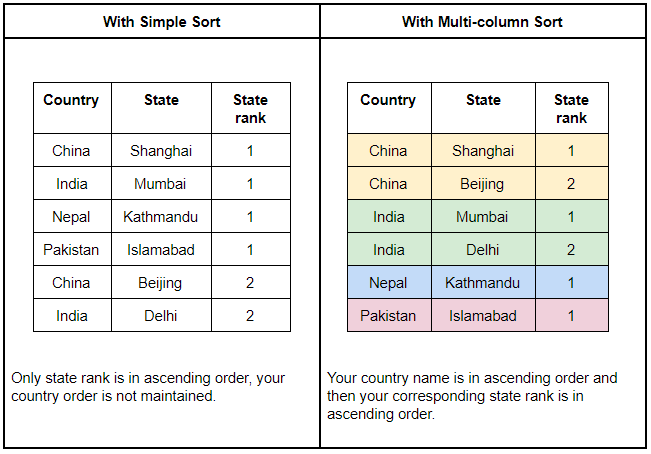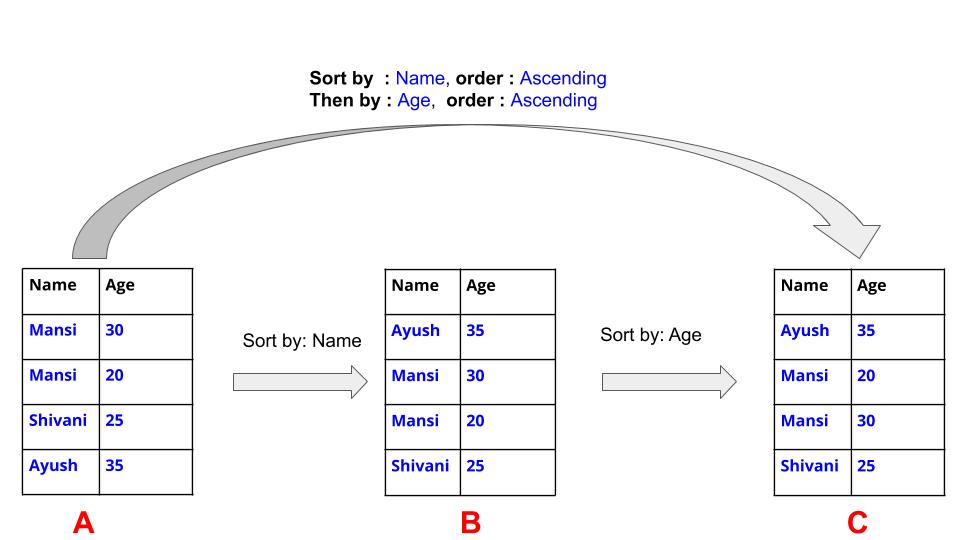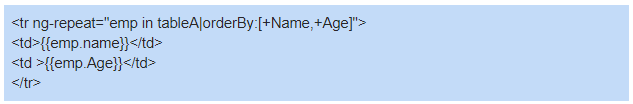You: Multi-column sort, why do we even want it? We have simple sorting
Me: Because it will give you simple sorting along with some extra feature
You: Which extra features?

What is a Multi-Column Sort?

Multi-column sort lets you sort columns one after another in such a way that the previous sort order does not get damaged by the next sort order.

Let’s understand it, with an example.

Let say you have this table:

 Country State State Rank India Mumbai 1 China Beijing 2 Pakistan Islamabad 1 Nepal Kathmandu 1 India Delhi 2 China Shanghai 1 Pakistan Lahore 2

Let’s say, you want a table with countries name in ascending order and with corresponding state rank in ascending order as well.

Therefore, in this operation:

• Sort by: Country, Order: Ascending and
• Then by: State rank, Order: Ascending

The results after applying the above operation would be.Hence, in sorting, we only consider one column value at a time but in multi-column sort, we can consider multiple column values at a time.

Note: In multi-column sort, a sort of order made previously must not get damaged by the next sort orders.

Where to use Multi-column sort?

Multi-column  Sort changes are visible when we have several duplicate values in the table. If we have unique values then we may not observe these changes.

I know it might seem little confusing, however, remember “In multi-column sort, a sort of order made previously must not get damaged by the next sort orders.”  Try to observe this in the table.

How to achieve multi-column sort in AngularJS?In AngularJS we have a filter “orderBy” and by using that we can implement multi-column sort easily.

For example:

In the table above, on “Table A” we can perform (​Sort by: Name, order: Ascending) and we can achieve the following:Then we get “Table B” as a result.

In “Table A” we can perform ​(​Sort by: Name, order: Ascending and Then by: Age, order: Ascending), by this we obtain:Hence, we get “Table C” as a result.

In the above cases, we have used the “+” sign for ascending order and “-” sign for descending order.

So, for implementing multi-column sort we simply have to push the column name into an array along with prefix, “+” and “-” as per the selected order, after which we assign that array value to “orderBy” filter.

Implementation of the multi-column sort in AngularJS

In the above sample,

1. You get to see a table and a button with name “multi-column sort”

2. When you click on button then modal (Dialogue box) opens

3. You can add or delete a row by clicking on “Add” and “Delete” button

4. On modal, you have to select the column name from the dropdown and corresponding order (we have implemented dropdown value in such way that the column you selected above will never appear in below dropdown)

5. Then click on Sort

6. Try to observe how values are changing.

For code visit my GitHub

To know how it is implemented, follow below steps. Every next step changes are highlighted.

Steps

1. The basic setup of the angular environment.

2. Create a table and pass values

3. Create a modal for column and order selection

4. Manage the dropdown values

5. Sort

Step 1: Create a html file (index.html) and JS file (script.js) as per AngularJS format.

index.html:

<!DOCTYPE html>
<html lang="en" >
<script src="./script.js"></script>
<body ng-app='myModule' >
<div ng-controller='myController'>
</div>

</body>
</html>

script.js

let app = angular.module("myModule",[]);
app.controller("myController", (\$scope) => { });

Step 2: Create a table and pass column name values in array and Table values in object type.

index.html:Script.js

let app = angular.module("myModule",[]);
var employees=[
{name:"shivani", category:0,salary:"15000",Age:30,weight:50},
{name:"ayush", category:0,salary:"1800",Age:20,weight:55},
{name:"ayush", category:2,salary:"18000",Age:60,weight:35},
{name:"mansi", category:1,salary:"2500",Age:23,weight:45},
{name:"mansi", category:1,salary:"25000",Age:13,weight:60},
{name:"deepanshi",category:1,salary:"15000",Age:34,weight:53},
{name:"deepanshi",category:1,salary:"150",Age:22,weight:41},
{name:"manoj", category:2,salary:"10000",Age:25,weight:45},
{name:"manoj", category:0,salary:"1000",Age:15,weight:56},
{name:"shivani", category:1,salary:"1500",Age:60,weight:34},
];
var columnValue= ['name','category','salary','Age','weight'];

app.controller("myController", (\$scope) => {
\$scope.employees=employees;
\$scope.multiplesort=columnValue;

});

Step 3: create a  multicolumn sort button to open the modal and create the modal

index.html:modal.html

<div>
<div class="modal-content">
<div class="modal-title">Sort Column</div>
<div
type="button"
ng-click="modalclose()"
class="close_bar"
data-dismiss="modal"
aria-label="Close"
>
<span aria-hidden="true">×</span>
</div>
</div>

<div class="modal-body">
<div class="feature-button">
<button
type="button"
>
>&nbsp;
<button
id="delete"
type="button"
ng-disabled="delete_sort_button"
ng-click="deleteRow()"
>
<i class="fa fa-minus"></i>Delete Sort
</button>
</div>
<div class="modal-table">
<table class="module-row" class="table">
<tr>
<th></th>
<th><div class="th-inner">Column</div></th>
<th><div class="th-inner">Order</div></th>
</tr>
<tbody>
<tr >
<td>SortBy</td>
<td>
<select
ng-init="elements[-1].col = data"
class="dropdown"
ng-model="elements[-1].col"
ng-change="onsortBychange(elements[-1].col,-1)"
ng-options="option for option in data"
></select>

</td>
<td>
<select class="dropdown" ng-model="elements[-1].order" >
<option value="asc">Ascending</option>
<option value="desc">Descending</option>
</select>
</td>
</tr>
<tr ng-repeat="element in elements">
<td>ThenBy</td>
<td>
<select
ng-init="element.col = element.values"
class="dropdown"
ng-change="onsortBychange(element.col,\$index)"
ng-model="element.col"
ng-options="option for option in element.values"
></select>
</td>
<td>
<select class="dropdown" ng-model="element.order"
>
<option value="asc">Ascending</option>
<option value="desc">Descending</option>
</select>
</td>
<td><i class="fa fa-trash" ng-click="delete_particularcol(element.col,\$index)"></i></td>
</tr>
</tbody>
</table>
</div>
</div>
<br />
<div class="modal-footer">
<button
type="button"
class="btn btn-secondary"
ng-click="modalclose()"
data-dismiss="modal"
>
Cancel</button
>&nbsp;
<button
type="button"
class="btn btn-primary"
ng-click="onclickSort()"
aria-disabled="false"
style="background-color: lightskyblue;"
>
Sort
</button>
</div>
</div>
</div>

Step 4: Inside the controller, we wright all function logic

1. For opening and closing the modal

2. For adding and deleting a row

3. For managing the dropdown

4. Sort

Script.js

app.controller("myController", (\$scope) => {
\$scope.employees=employees;
\$scope.multiplesort=columnValue;
\$scope.MultipleSort_Popup = function () {
\$scope.modalOpen=!\$scope.modalOpen;
}

\$scope.sortColumn=[]
\$scope.sortColumn[i]={ col: "", order: "asc", values: [] };
});
// -------------------------------

\$scope.index = 0;
\$scope.elements = [];
\$scope.dataselected = [];
\$scope.result = [] = [{ col: "", order: "asc" }];
\$scope.elements[-1] = \$scope.sortColumn[-1]
= {
col: "",
order: "asc",
values: \$scope.data,
};
\$scope.delete_sort_button = true;
// ------------------------------------

\$scope.delete_sort_button = false;
if (\$scope.elements.length <= 0)
\$scope.dataselected.push(\$scope.elements[-1].col);
else
\$scope.dataselected.push(
\$scope.elements[\$scope.elements.length - 1].col
);
if (\$scope.elements.length < \$scope.data.length - 1) {
\$scope.sortColumn[\$scope.index].values = \$scope.data.filter(
(item) => !\$scope.dataselected.some((other) => item == other)
);
\$scope.sortColumn[\$scope.index].col =
\$scope.sortColumn[\$scope.index].values;
\$scope.elements.push(\$scope.sortColumn[\$scope.index]);
}
if (\$scope.elements.length == \$scope.data.length - 1)
\$scope.index++;
};
// --------------------------------
\$scope.deleteRow = function () {
\$scope.elements.pop();
\$scope.sortColumn[\$scope.elements.length] = {
col: "",
order: "asc",
values: [],
};
\$scope.index--;
\$scope.dataselected.pop();
if (\$scope.elements.length <= 0) \$scope.delete_sort_button = true;
};
// ---------------------------------------------------
\$scope.onsortBychange = function (changeval, changedindex) {
if (changedindex == \$scope.elements.length - 1) return;
let prevval = \$scope.dataselected[changedindex + 1];
for (let i = changedindex + 1; i < \$scope.elements.length; i++) {
var position = \$scope.elements[i].values.indexOf(changeval);
\$scope.elements[i].values[position] = prevval;
if (\$scope.elements[i].col == changeval) {
\$scope.elements[i].col = prevval;
}
}
let changeval_dataselected = \$scope.dataselected.indexOf(changeval);
if (changeval_dataselected > -1)
\$scope.dataselected[changeval_dataselected] = prevval;
\$scope.dataselected[changedindex + 1] = changeval;
return;
};
// -------------------------------
\$scope.delete_particularcol = function (delval, delindex) {
if (delindex == \$scope.elements.length - 1) {
\$scope.elements.pop();
\$scope.sortColumn[\$scope.elements.length] = {
col: "",
order: "asc",
values: [],
};
\$scope.index--;
} else {
for (let i = delindex + 1; i < \$scope.elements.length; i++) {
\$scope.elements[i].values.push(delval);
}
\$scope.elements.splice(delindex, 1);
let position = \$scope.dataselected.indexOf(delval);
\$scope.dataselected.splice(position, 1);
\$scope.sortColumn[\$scope.elements.length] = {
col: "",
order: "asc",
values: [],
};
\$scope.index--;
}};
// -------------------------
\$scope.onclickSort = function () {
\$scope.result = {
col: \$scope.elements[-1].col,
order: \$scope.elements[-1].order,
};
for (let i = 1; i <= \$scope.elements.length; i++) {
\$scope.result[i] = {
col: \$scope.elements[i - 1].col,
order: \$scope.elements[i - 1].order,
};
}
\$scope.modalOpen=false;
console.log(JSON.stringify(\$scope.result));
\$scope.chosen_multisort=[];
for(let i=0;i<\$scope.result.length;i++){
if(\$scope.result[i].order==="asc")
\$scope.chosen_multisort.push('+'+\$scope.result[i].col);
else if(d3ata[i].order==="desc")
\$scope.chosen_multisort.push('-'+\$scope.result[i].col);
}
console.log(JSON.stringify(\$scope.chosen_multisort));
};
// ------------------------
\$scope.modalclose = function () {
\$scope.modalOpen=false; };

});

Step 5: Finally add the sorted column array with sign-in “orderBy” filter

index.html:References: Modal look

AngularJS has now been around for quite some time and has gained notable acclaim in the web development domain. AngularJS is apt for web enterprise development. This front end framework's focus mainly lies on code quality and testability, making it great for developers. Want to hire Angular Developers? Do visit us today.

Want to work abroad? Check out jobs in the USA here.